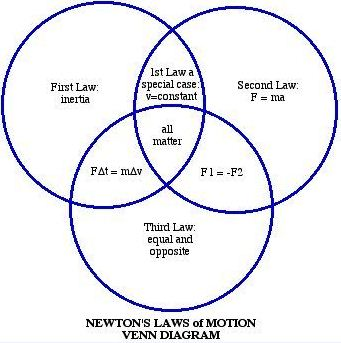### Tech & Space

﻿# Important Laws of Physics

Physical laws are the conclusions drawn on the basis of long year scientific observations and experiments which are repeated again and again under different conditions to reach the assumptions which can be accepted worldwide. We all know our world works on some principles and these principles are drawn by our scientists in the form of certain physical laws. Let us discuss some important laws of physics which will enhance knowledge as well as help in the preparation of various entrance examinations.

Important Laws of Physics

1. Archimedes Principle : The principle was discovered in 3rd century B.C. by the Greek mathematician. Archimedes. It states that when a body is partially or totally immersed in a fluid, it experiences an upward thrust equal to the weight of the fluid displaced by it that i.e. its apparent loss of weight is equal to the weight of liquid displaced.
2. Avagadro’s Law : In 1811 it was discovered by an Italian Scientist Anedeos Avagadro. This law states that equal volume of all gases under the same conditions of temperature and pressure contain equal number of molecules.
3. Ohm's Law : It states that the current passing through a conductor between two points is directly proportional to the potential difference across the two points provided the physical state and temperature etc. of the conductor does not change.
4. Newton’s Laws (1642-1727) : Let us begin our explanation of how Newton changed our understanding of the Universe by enumerating his Three Laws of Motion.Law of Gravitation: Objects attract each other with a force directly proportional to the product of the masses of the objects and inversely proportional to the square of the distance between them. Hence, for objects on or near the earth, the mass of the earth is very much greater than the object, and so the gravitational force between them makes objects fall towards the earth. That is why lead and feather fall at the same rate in a vacuum.

• Newton's First Law of Motion: Every object in a state of uniform motion tends to remain in that state of motion unless an external force is applied to it.
• Newton's Second Law of Motion:  The relationship between an object's mass m, its acceleration a, and the applied force F is F = ma. Acceleration and force are vectors (as indicated by their symbols being displayed in slant bold font); in this law the direction of the force vector is the same as the direction of the acceleration vector.
• Newton's Third Law of Motion: For every action there is an equal and opposite reaction. This is the principle behind the recoil felt on pulling the trigger of a gun.

Newton’s Law of cooling
The rate at which a body cools or loses its heat to its surroundings is proportional to the excess of mean temperature of the body over that of the surroundings, provided this temperature excess is not too large.

5. Coulomb’s Law (1738-1806) : The force between the two electric charges reduces to a quarter of its former value when the distance between          them is doubled. The SI unit of electric charge, coulomb, is named after Charles Augustin de Coulomb who established the law.

6. Stefan’s Law (1835-1883) : The total energy radiated from a black body is equal to the fourth power of its absolute temperature.

7. Pascal’s Law (1623-1662) : When pressure is applied to a fluid, the pressure change is transmitted to every part of the fluid without loss.                  Hydraulic machines like the hydraulic press work on this principle.
Atmospheric pressure decreases with increase in height. The SI unit of pressure is pascal which is named after Pascal who established this law.

8. Hooke’s Law (1635-1703) : This law states that the extension of a spring is proportional to the tension stretching it. Doubling of the tension                results in the doubling of the amount of stretch.

9. Bernoulli's Principle : It states that as the speed of a moving fluid, liquid or gas, increases, the pressure within the fluid decreases. The                    aerodynamic lift on the wing of an aeroplane is also explained in part by this principle.

10. Boyles's Law : It states that temperature remaining constant, volume of a given mass of a gas varies inversely with the pressure of the gas.

11. Charles's Law : It states that pressure remaining constant, the volume of a given mass of gas increases or decreases by 1/273 part of its                  volume at 0 degree Celsius for each degree Celsius rise or fall of its temperature.

12. Kepler's Law : Each planet revolves round the Sun in an elliptical orbit with the Sun at one focus. The straight line joining the Sun and the                planet sweeps out equal areas in equal intervals. The squares of the orbital periods of planets are proportional to the cubes of their mean                  distance from the Sun.

13. Law of conservation of energy : It states that energy can neither be created nor destroyed but it can be transformed from one form to                      another. Since energy cannot be created or destroyed, the amount of energy present in the universe is always remain constant.

14. Tyndall effect : The scattering of light by very small particles suspended in a gas or liquid.

15. Graham’s Law : It states that the rates of diffusion of gases are inversely proportional to the square roots of their densities under similar                    conditions of temperature and pressure.

We have 158 guests and no members online